# NTSE Sample Questions 2016-17 : Tamil Nadu SAT (Stage-1)

Disclaimer: This website is NOT associated with CBSE, for official website of CBSE visit - www.cbse.gov.in

#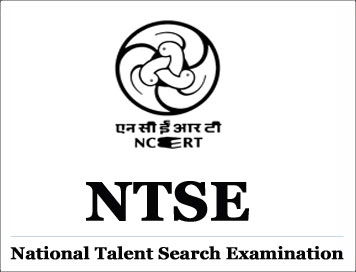## NTSE Sample Questions 2016-17 : Tamil Nadu SAT (Stage-1)

101. When 10 x2 + x – 23 is divided by (2x + 3), the reminder is:
(A) 1 (B) -2 (C) 2 (D) 0

102. If  α and β are the zeros of the polynomial 25 x2 - 16, then α2 + β2 is: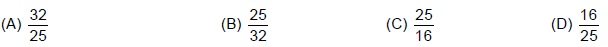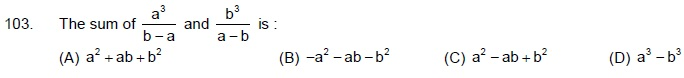104. Sum of the digits of two digit number is 9. The number obtained by interchanging the digits is 18 more than twice the original number. The original number is:
(A) 72 (B) 27 (C) 36 (D) 63

105. Which of the following are irrational numbers?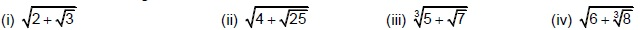(A) (i), (ii) (B) (iii), (iv) (C) (i), (iii) (D) (iv), (iv)

106. For which value, point A(a, b) lies in the quadrant III:
(A) a > 0, b < 0 (B) a < 0, b < 0 (C) a > 0, b > 0 (D) a < 0, b > 0
107. If the LCM of 12 and 42 is (10 m + 4) then the value of ‘m’ is:
(A) 50 (B) 8 (C) 1/5 (D) 1

108. If the perimeter of protractor is 72 cm, then it’s radius is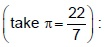(A) 7 cm (B) 21 cm (C) 14 cm (D) 3.5 cm

109. The degree of the polynomial (x + 1) (x2 -x - x4 + 1) is:
(A) 2 (B) 3 (C) 4 (D) 5

110. Two right circular cones have same radii. Ratio of their slant height is 4: 3, then the ratio of their curved surface areas is:
(A) 16: 9 (B) 2: 3 (C) 4: 3 (D) 3: 4

111. AB and CD are two chords of a circle which intersect each other externally at p. if AB = 4 cm, BP = 5 cm , PD = 3 cm, then the length of CD is:
(A) 10 cm (B) 12 cm (C) 8 cm (D) 11 cm

112. The radii of two concentric circles are 7 cm and 14 cm are respectively. The area between the two sectors of the circles whose central angle 60° is:
(A) 154 sq. cm (B) 77 sq. cm (C) 308 sq. cm (D) 98 sq. cm

113. Arithmetic mean of 20 observations is 15. if each observation is multiplied by2/3 then the arithmetic mean of them is:
(A) 10 (B) 30 (C) 45 (D) 15

114. There are 6 defective items in a sample of 20 items. One items is drawn at random. The probability that it is a non – defective item is:
(A) 7/10 (B) 0 (C) 3/10 (D) 2/3

115. Segment of a quadrant of a circle has area equal to: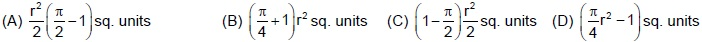116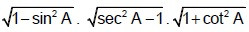(A) 0 (B) 2 (C) 1 (D) -2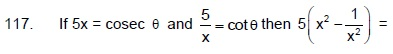(A) 25 (B) 1 (C) 1/5 (D) -5

118. If x = a cos θ , y = sin θ , then x2+y2 =
(A) 1 (B) a (C) 2 a (D)  2 2 a